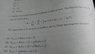# Coupled 2D harmonic oscillators

• Apashanka das

#### Apashanka das

1. The problem statementhttps://www.physicsforums.com/attachments/225935

## Homework Equations

3. I have rescaled coordinates which are X=(x1+x2)/√2 and Y=√3(x1-x2)/√2 for which the potential term becomes for a 2D harmonic oscillator of coordinates X and Y. But how to express Kinetic terms in terms of these new coordinates X and Y?

#### Attachments

•P_20180519_195615.jpg
30.9 KB · Views: 427
Last edited:

solve for $x_1$ and $x_2$ in terms of $X, Y$ then find the kinetic energy.

solve for $x_1$ and $x_2$ in terms of $X, Y$ then find the kinetic energy.
sorry sir I didn't get you here QM kinetic term is nedded

$\hat{p}$ can be related to the $\dot{X}$, you just have to find the momentum operators in terms of your new coordinates.

$\hat{p}$ can be related to the $\dot{X}$, you just have to find the momentum operators in terms of your new coordinates.
sir but in calculating p2 there will be again interaction term between X And Y
Sir I am actually trying to find d2/dx12 in terms of d2/dX2 and d2/dY2

$\hat{p}$ can be related to the $\dot{X}$, you just have to find the momentum operators in terms of your new coordinates.
O thank you sir I have got it
The ans is option B